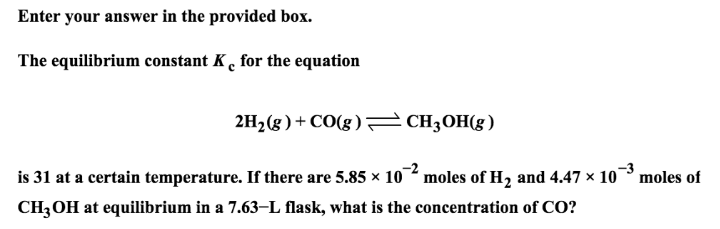# Problem: Enter your answer in the provided box.The equilibrium constant Kc for the equation 2H2 (g) + CO (g) ⇌ CH3OH (g) is 31 at a certain temperature. If there are 5.85 x 10-2 moles of H2 and 4.47 x 10-3 moles of CH3OH at equilibrium in a 7.63-L flask, what is the concentration of CO?

###### FREE Expert Solution
84% (489 ratings)###### Problem Details

The equilibrium constant Kc for the equation

2H2 (g) + CO (g) ⇌ CH3OH (g)

is 31 at a certain temperature. If there are 5.85 x 10-2 moles of H2 and 4.47 x 10-3 moles of CH3OH at equilibrium in a 7.63-L flask, what is the concentration of CO?